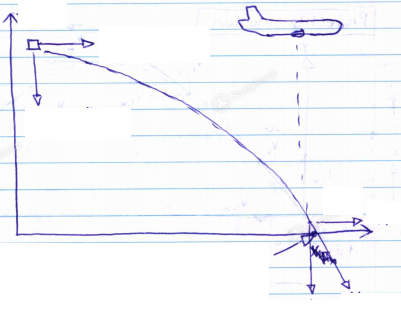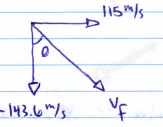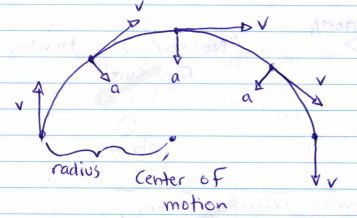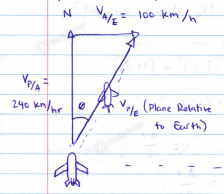×
Get Full Access to GVSU - PHY 230 - Class Notes - Week 6
Get Full Access to GVSU - PHY 230 - Class Notes - Week 6

×

GVSU / Physics / PHYS 230 / What is the definition of velocity?

# What is the definition of velocity? Description

##### Description: Covers: - Projectile motion - Circular motion - Relative motion - Newton's First Law
10 Pages 205 Views 1 Unlocks
Reviews

Example 1 Projectile Motion

A plane at a constant altitude of 1050 m and traveling at a speed of 115 m/s in the +x direction drops a crate that plummets to the Earth. Determine final velocity, total time, and final x position. Assume +y is upwards and +x is parallel to the plane's initial velocity. Don't forget about the age old question of What should reactants have for reactions to occur?

Givens                                        Picture+yWe also discuss several other topics like a mental shortcut that helps us to streamline our thinkingDon't forget about the age old question of Can our senses be detectives?If you want to learn more check out What does the suffix -phil mean?
We also discuss several other topics like What is the standard formula in solving for arc length?Don't forget about the age old question of What are the two classes of drug laws?+ x1) Between the x and y directions we know that one variable will remain the same time.

So first solve for time.

2) More Y components are known than X, so let's solve for t in the y direction.3) Now that we know t, we can solve for4) To find, we must use its components.

We already know, so we must calculate5) Use to solve for. Pythagorean theorem.Circular Motion

⤷ a special case in 2D motion.

Velocity Tangent to circle/arc of motion and constantly changing direction

Acceleration Perpendicular to circle/arc of motion toward the centerRelative Motion (1D)

When there are multiple observers, we often indicate the reference frame in the subscript.→ velocity of object A relative to observer B

Ex. Consider an observer in a vehicle and an observer on the road, both observing another car.

Car A ⇒ 40 m/s        “I think car B is moving at 30 m/s”

Car B ⇒ 70 m/s        “No way! It’s traveling 70 m/s for sure!”

|

O

Observer

Converting between reference framesExample 1

A plane is headed North according to its compass and flies with an airspeed of 240 km/hr

A cross wind at 100 km/h from west to east pushes the plane sidewaysVelocity of the plane relative to earth isPage ExpiredIt looks like your free minutes have expired! Lucky for you we have all the content you need, just sign up here